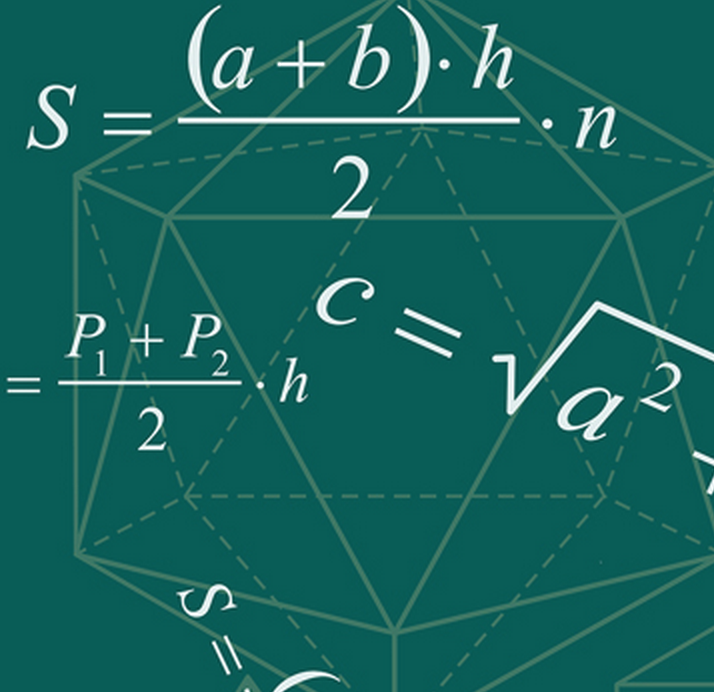# ACT & SAT Prep and College Admissions BlogThe SAT sometimes approaches math a bit differently than we’re used to in math class.  One of these differences in approach is in the way some SAT math problems ask us to solve for relationships between variables, rather than the value of one particular variable.  These problems can seem very challenging, or even downright impossible, at first glance, because they often give us multiple variables but only one equation.

One thing we learn in math class when studying systems of equations is that in order to solve for 2 variables, we usually need 2 separate equations; to solve for 3 variables, we need 3 separate equations; and so on.   Certain SAT math problems will appear to violate that general rule and ask us to do something that’s supposed to be impossible, like solving for 2 variables with only 1 equation.  When we look more closely at such problems, though, we realize that the SAT is not really asking for the value of each individual variable involved.  Instead, we’re asked to find the value of the sum, product, or some other relationship involving 2 or more variables.

Let’s look at an example of such a problem:

If 4x + 7y + 1 = y – 2,

What is the value of 2x + 3y?

A.  -2

B.  -1.5

C.  -0.5

D.  2

E.  4.5

Explanation and solution:  We’re given only one equation, but it involves 2 unknowns:  x and y.  We really don’t have enough information to solve for the value of x and/or the value of y, but fortunately, that’s not what the question asks us to do.  Instead, we are asked to find the value of a sort of relationship that involves both x and y together:  2x + 3y.  To do this, we algebraically manipulate the equation that’s given.  Instead of trying to isolate x or isolate y, we try to isolate the quantity “2x + 3y”.  To do this, we can first subtract y from both sides of the equation, and then subtract 1 from both sides, so that the equation now looks like:  4x + 6y = -3.   To finish isolating “2x + 3y”, we divide both sides of the equation by 2 and obtain:  2x + 3y = -1.5.  So the correct answer choice is “B”.

Let’s look at another example:

If the average of x, y, and z is 12,  what is the value of 3(x + y + z)?

A.  12

B.  24

C.  36

D.  72

E.  108

Explanation and solution:  To obtain the average of x, y, and z, we would need to add the 3 numbers together and then divide the resulting sum by 3.  Since we are told that their average equals 12, we can express the information as an equation:

(x + y + z)/3 = 12.   Multiplying both sides of the equation by 3, we obtain:  (x + y + z) = 36.  To find the value of 3(x + y + z), we can again multiply both sides of the equation by 3 and find that 3(x + y + z) = 108.  The correct answer choice is “E”.

Notice that in the problem we just solved, we did not solve for the individual values of x, y, or z; that would have required more information than we were given.Topics: SAT, math skills